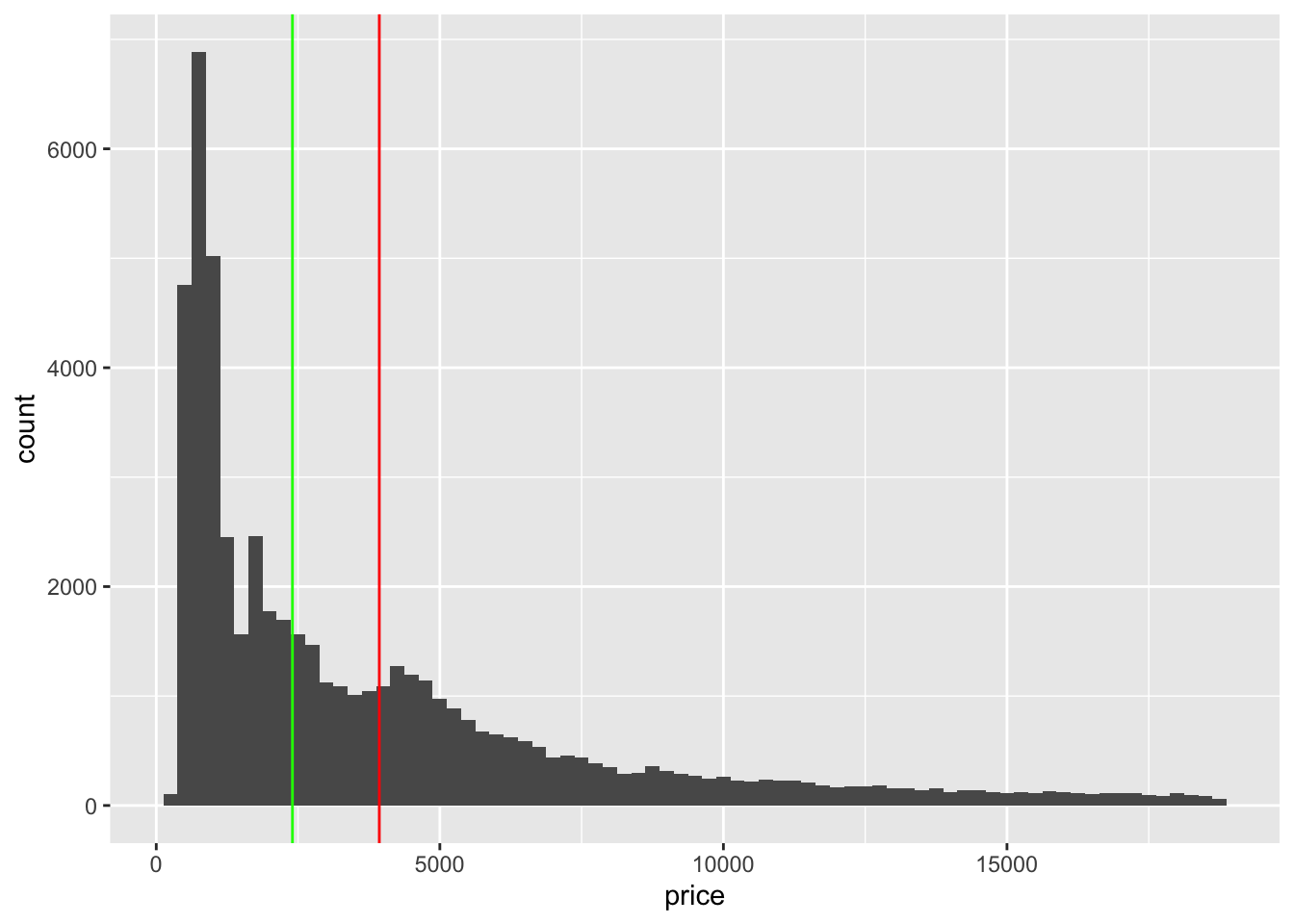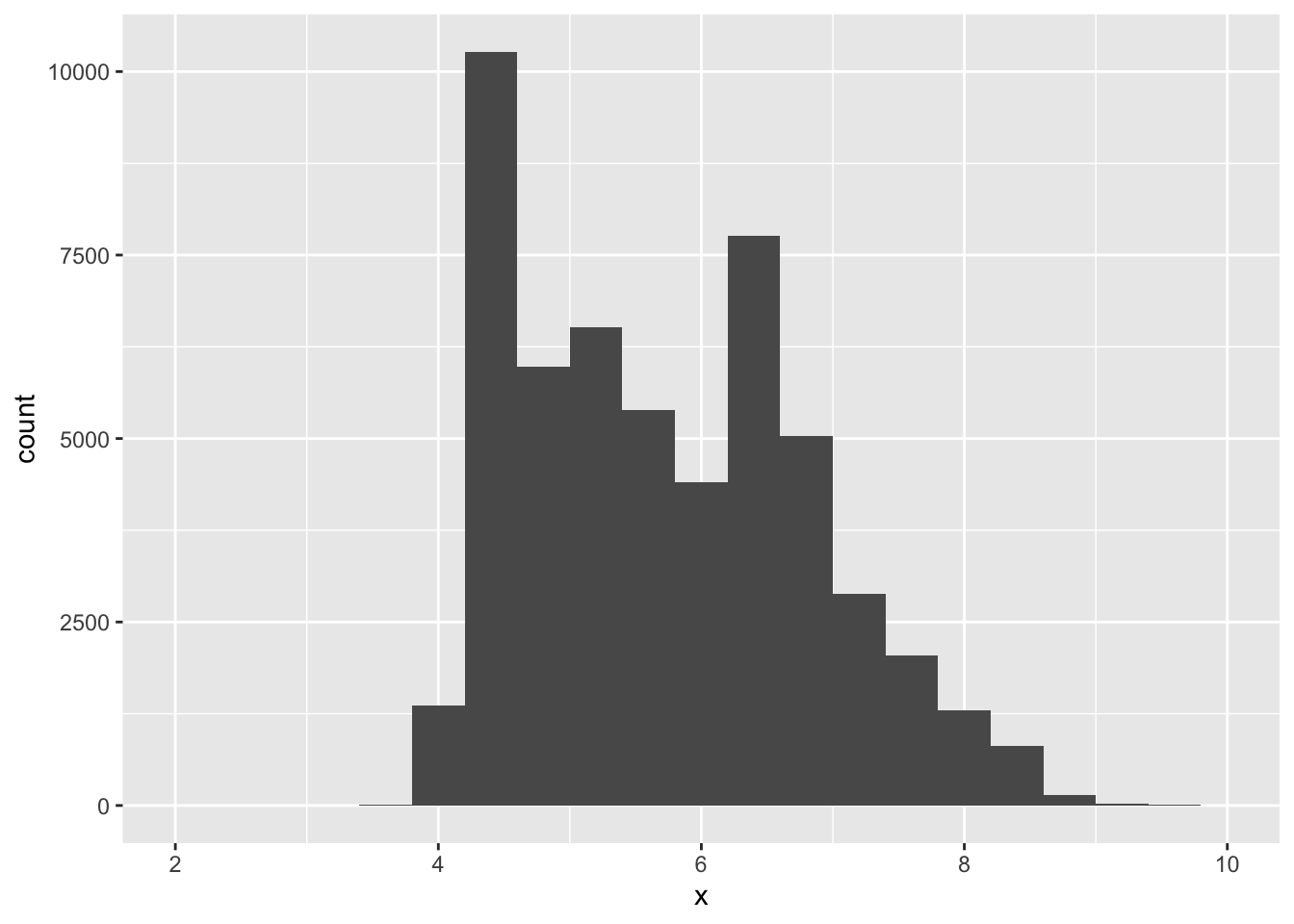1. What tidyverse function would you use to extract rows?

a. filter()

1. count()
2. select()
3. distinct()
1. What tidyverse function would you use to extract colums?
1. filter()
2. count()
3. c. select()
4. distinct()
1. To find the maximum number of times that a categorical variable appears which function should I use?
1. filter()
2. b. count()
3. select()
4. distinct()
1. What does the “%>%” operator do?

a. used in tidyverse between layers of dplyr coding

b. used in ggplot to add more layers of coding

1. Will this code run without an error? diamonds %>% mutate(price_hundred = price %/% 100)
1. It will produce an error
2. b. It will not produce an error
1. What does the binwidth input in ggplot do?
1. It controls the opacity of the plot
2. It can be used to update the labels
3. c. It controls the smoothness of a density plot
4. It controls the size of dots
1. What punctuation must be placed before a function or dataset to access more information?
1. “.”
2. “+”
3. c. “?”
4. “!”
1. Where should the variable names go in the following line of code: ggplot(data = A) + B(mapping = aes(C))
1. A
2. B
3. c. C
1. What does the here package do?
1. for data manipulation and display
2. b. to organize files
3. none of the above
1. Which of the following variables are discrete?

a. number of employees in a company.

1. distance traveled to and from work
2. taxes paid in 2019
3. purchases from 2018
1. What is the difference between distinct() and count()?
1. they are the same
2. b. distinct() gives the levels in a variable, count() gives the marginal distribution
3. distinct() gives the marginal distribution, count() gives the levels in a variable
1. What operator is used in ggplot?
1. “.”
2. b. “+”
3. “?”
4. “!”

Categorical variables

1. Find the expected count for clarity VS1 and color I for a $$\chi^2$$ distribution assuming independence.

$$8171\times 5422/53940$$

# A tibble: 8 × 10
clarity     D     E     F     G     H     I     J marginal_clarity marginal_…¹
<ord>   <int> <int> <int> <int> <int> <int> <int>            <dbl>       <dbl>
1 I1         42   102   143   150   162    92    50              741        6775
2 SI2      1370  1713  1609  1548  1563   912   479             9194        9797
3 SI1      2083  2426  2131  1976  2275  1424   750            13065        9542
4 VS2      1697  2470  2201  2347  1643  1169   731            12258       11292
5 VS1       705  1281  1364  2148  1169   962   542             8171        8304
6 VVS2      553   991   975  1443   608   365   131             5066        5422
7 VVS1      252   656   734   999   585   355    74             3655        2808
8 IF         73   158   385   681   299   143    51             1790       53940
# … with abbreviated variable name ¹​marginal_color
 821.3415
1. What proportion of the very good cut diamonds are colored I?

0.0997

# A tibble: 5 × 8
cut            D      E      F      G      H      I      J
<ord>      <dbl>  <dbl>  <dbl>  <dbl>  <dbl>  <dbl>  <dbl>
1 Fair      0.0241 0.0229 0.0327 0.0278 0.0365 0.0323 0.0424
2 Good      0.0977 0.0952 0.0953 0.0771 0.0845 0.0963 0.109
3 Very Good 0.223  0.245  0.227  0.204  0.220  0.222  0.241
4 Premium   0.237  0.239  0.244  0.259  0.284  0.263  0.288
5 Ideal     0.418  0.398  0.401  0.433  0.375  0.386  0.319 
# A tibble: 5 × 8
# Groups:   cut 
cut           D     E     F     G     H      I      J
<ord>     <dbl> <dbl> <dbl> <dbl> <dbl>  <dbl>  <dbl>
1 Fair      0.101 0.139 0.194 0.195 0.188 0.109  0.0739
2 Good      0.135 0.190 0.185 0.178 0.143 0.106  0.0626
3 Very Good 0.125 0.199 0.179 0.190 0.151 0.0997 0.0561
4 Premium   0.116 0.169 0.169 0.212 0.171 0.104  0.0586
5 Ideal     0.132 0.181 0.178 0.227 0.145 0.0971 0.0416
1. Which of the lines below is the mean, median, or mode?1. red is median, green is mean
2. red is mean, green is mode
3. red is mode, green is mean
4. d. red is mean, green is median
1. Is the mean or median a better measure of center in this distribution.

The mean is a better estimate in a a symmetric distribution, median in a skewed distribution1. Which plot is best at displaying outliers:
1. histogram
2. dotplot
3. plot of proportions
4. d. boxplot